# Hardest colleges to get into in Georgia

Top 10 colleges in Georgia with the lowest acceptance rates
What are the hardest colleges to get into in Georgia? We've got you covered. We've compiled a national college database and have created a list of the hardest universities to get into in Georgia below. These are the hardest 4 year colleges to get into in Georgia, and sometimes some of the hardest colleges to get into in the US. You could even say these are the best colleges in Georgia. We also include each college's average SAT and ACT scores so that you can see where you're most competitive. Read on to find out more.

## Emory University acceptance rate

Emory University acceptance rate is 18.5%.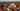The average SAT score for Emory University is 1435.

• SAT composite: 1435
• SAT math: 740

The average ACT score for Emory University is 33.

## Georgia Institute of Technology acceptance rate

Georgia Institute of Technology acceptance rate is 22.6%.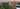The average SAT score for Georgia Institute of Technology is 1465.

• SAT composite: 1465
• SAT math: 750

The average ACT score for Georgia Institute of Technology is 33.

## Paine College acceptance rate

Paine College acceptance rate is 32%.The average SAT score for Paine College is 830.

• SAT composite: 830
• SAT math: 410

The average ACT score for Paine College is 16.

## Spelman College acceptance rate

Spelman College acceptance rate is 43.4%.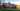The average SAT score for Spelman College is 1155.

• SAT composite: 1155
• SAT math: 560

The average ACT score for Spelman College is 24.

## Clayton State University acceptance rate

Clayton State University acceptance rate is 48%.The average SAT score for Clayton State University is 951.

• SAT composite: 951
• SAT math: 460

The average ACT score for Clayton State University is 20.

## University of Georgia acceptance rate

University of Georgia acceptance rate is 48.6%.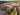The average SAT score for University of Georgia is 1325.

• SAT composite: 1325
• SAT math: 660

The average ACT score for University of Georgia is 30.

## Columbus State University acceptance rate

Columbus State University acceptance rate is 53.4%.The average SAT score for Columbus State University is 978.

• SAT composite: 978
• SAT math: 480

The average ACT score for Columbus State University is 21.

## Georgia State University acceptance rate

Georgia State University acceptance rate is 57.4%.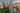The average SAT score for Georgia State University is 1090.

• SAT composite: 1090
• SAT math: 545

The average ACT score for Georgia State University is 24.

## Kennesaw State University acceptance rate

Kennesaw State University acceptance rate is 57.9%.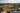The average SAT score for Kennesaw State University is 1175.

• SAT composite: 1175
• SAT math: 580

The average ACT score for Kennesaw State University is 24.

## University of West Georgia acceptance rate

University of West Georgia acceptance rate is 58.6%.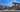The average SAT score for University of West Georgia is 1000.

• SAT composite: 1000
• SAT math: 470